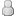• 0 Votes - 0 Average
• 1
• 2
• 3
• 4
• 5
 Annual rate of return and risk of assets
02-11-2010, 02:48 PM
Post: #1
 hinanifafJunior MemberPosts: 35 Joined: Aug 2009 Reputation: 0
Annual rate of return and risk of assets
Good Day colleqs
This are the annual cash flows and beginning and end-of-year values of each asset over the immediately preceding 3years.
<u>Principles of Managerial Finance - By Lawrence J. Gitman </u>
<u>Return Data for Assets A and B 2000 - 2002</u>
Asset A Asset B
Year Cashflow Begin Ending Cashflow Begin Ending
2000 1000 20000 22000 1500 20000 20000
2001 1500 22000 21000 1600 20000 20000
2002 1400 21000 24000 1700 20000 21000

Each asset's risk can be assessed in two waysin isolation and as part of the firm's diversified portfolio of assets.STD deviation & coefficient of variation of returns can be used to find the risk of assets in isolation.CAPM can be use to assess the assets risk as part of the firm's portfolio of assets.Estimated betas for assets A & B is 1.60 & 1.10 respectively.Risk-free rate is currently 7% and the market return is 10%.
<u>Question </u> is what will be the annual rate of return for each asset in each of the 3preceding years,than use those values to find the average annual rate of return for each asset over the 3yr.Using those returns to calculate the risk of those returns for each asset over the 3yrs.
02-19-2010, 04:06 AM
Post: #2
 june23amMemberPosts: 57 Joined: Oct 2009 Reputation: 0

first to find <b>annual rate of return</b>, for which formula is.. (ending valuePt-beginning valuePt-1 + cash flowCt) / begining valuePt-1
<b>for asset a</b>
year cashflow(Ct) beginingvalue(Pt-1) endingvalue(Pt)
2000 1000 20000 22000 so 22000-20000+1000/20000 *100 which will be 15% with a gain of 2000
year 2001
21000-22000+1500/22000 *100 which will be 2.27% with loss of 1000
year 2002
24000-21000+1400/21000 *100 which will be 20.95% with 3000 gain
average annual return for asset a is 12.74 the average of (2.27+20.95+15/3)
<b>for asset b</b>
year 2000
20000-20000+1500/20000 *100 which is 7.50% with 0 gain/loss
year 2001
20000-20000+1600/20000 *100 which is 8.00% with 0 gain/loss
year 2002
21000-20000+1700/20000 *100 which is 13.50 with 1000 gain
average annual return for asset b is 9.67 average of (13.50+8.00+7.50%/3)
this is how anual rate of return and average annual rate of return will be calculated,
i dont understand this line of yours "<b>Using those returns to calculate the risk of those returns for each asset over the 3yrs</b>." clear it so i can further help you with this if you want to. jazakALLAH
 « Next Oldest | Next Newest »

Forum Jump:

User(s) browsing this thread: 1 Guest(s)